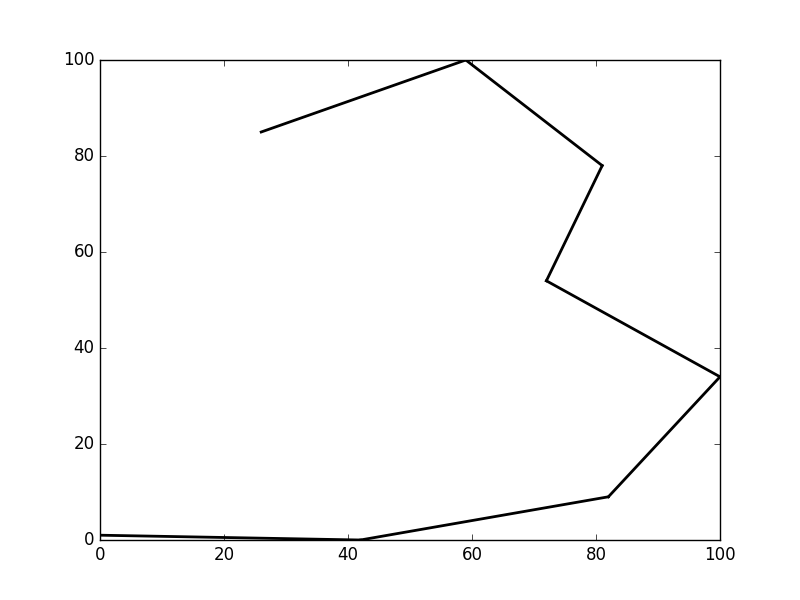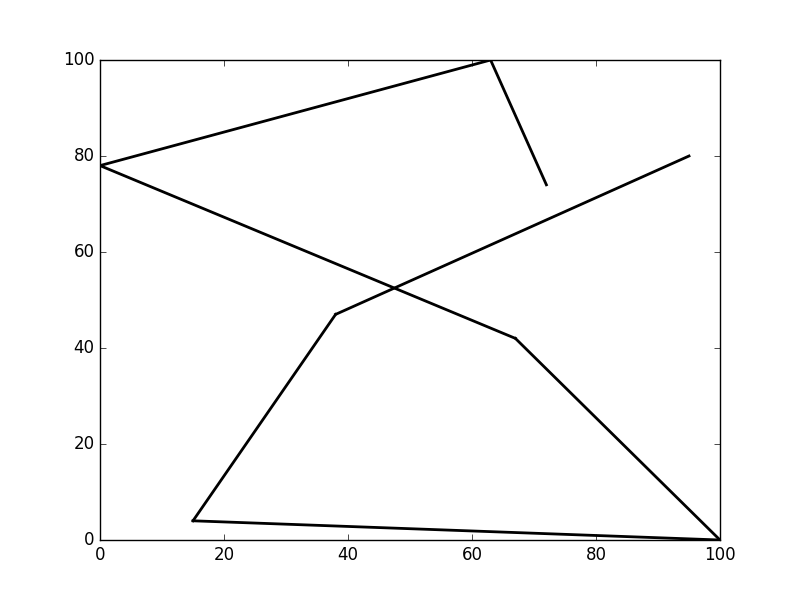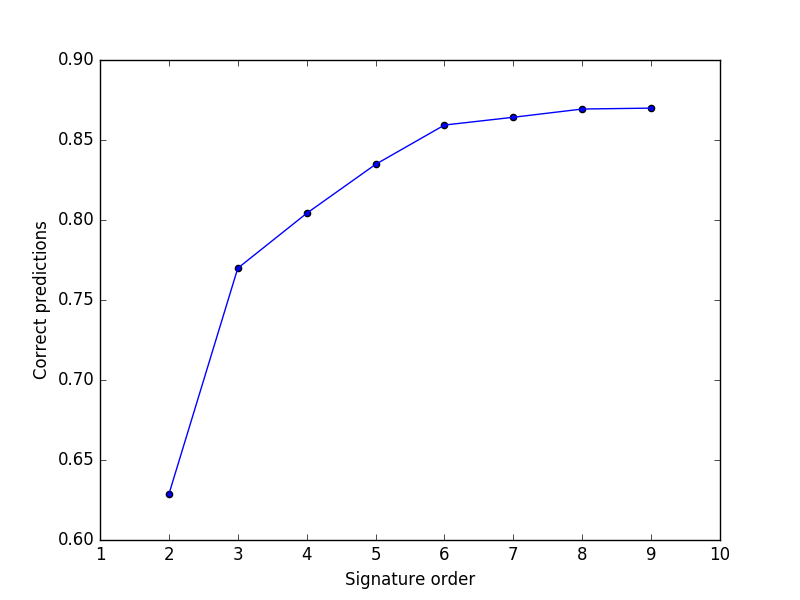Rough Path Theory and Signatures Applied To Quantitative Finance - Part 3

Rough Path Theory and Signatures Applied To Quantitative Finance - Part 3

This is the third in a new advanced series of posts written by Imanol Pérez, a PhD researcher in Mathematics at Oxford University and an expert guest contributor to QuantStart.

In this post Imanol applies the Theory of Rough Paths to the task of handwritten digit classification—a common task for testing the effectiveness of machine learning models.
- Mike.

As we discussed in the last article, signatures enjoy many properties that can be exploited to create a signature-based machine learning model. In that article a Python package was created to apply this model to concrete examples. In this article, the package will be used to show the power of signatures.

Handwritten digit recognition is quite a popular challenge in machine learning and is often used to test the performance of machine learning models. Thanks to its popularity there are many free databases that can be downloaded. We will first test our model using one of these databases in order to show that the signature-based model performs well.

Handwritten digits can be seen as continuous paths in a straightforward way and we can therefore calculate their signature. As we will see the signature of a handwritten digit will be distinctive enough to distinguish digits from each other.

For our task we will use the database from the UCI repository. The dataset contains handwritten digit samples from 44 different writers. Each of the samples consists of 17 numbers in the following format:

where $(x_i, y_i)$ are points on the plane, with $0 \leq x_i, y_i \leq 100$, and $D$ is the digit (an integer from $0$ to $9$) that corresponds to the sample. Each sample was obtained from a writer that uses a digital pen to write on a tablet. The tablet records the coordinates of the pen at 8 different time points. The objective is to use this information to predict the digit $D$.

There are 10992 samples in the database in total. The dataset is divided into a training set of 7494 samples and a testing set of 3498 samples.Fig 1a - Digit 3Fig 1b - Digit 8

Using Python and the package created in the last article in particular, we will create a model that uses the dataset from the UCI repository to recognise handwritten digits. The complete code can be found in this Git repository.

First we will create a simple class to store information about a digit.

class Digit:
def __init__(self, points, digit):
self.points=points
self.digit=digit


We will use this class to store the stream of data of each digit, as well as the actual digit it comes from. We will now load the dataset. This will be done using the Python2 package urllib2. Taking into account the format of the dataset, which was discussed earlier, we can use the following function:

def loadDigits(path):
data = urllib2.urlopen(path)
digits=[]
for line in data:
digitList=line.replace(",", " ").replace("\n", "").split()
number=float(digitList)
points=np.array([[float(digitList[i]), float(digitList[i+1])]
for i in range(0,len(digitList)-1, 2)])
digit=Digit(points, number)
digits.append(digit)

return digits


The function returns a list of objects of class Digit. We will use this function to load the training set and extract the inputs (streams of data) and outputs (the actual digit that has to be predicted) from it:

trainingPath="https://archive.ics.uci.edu/ml/
machine-learning-databases/pendigits/pendigits.tra"
inputs=[digit.points for digit in trainingSet]
outputs=[digit.digit for digit in trainingSet]


Now, using the package created in the last article, we create and train our model (in this example, we use the 5th order signature):

model=sigLearn.sigLearn(order=5)
model.train(inputs, outputs)


After the model is trained we can use the testing set to make predictions:

testingPath="https://archive.ics.uci.edu/ml/
machine-learning-databases/pendigits/pendigits.tes"

testingInputs=[digit.points for digit in testingSet]
testingOutputs=[digit.digit for digit in testingSet]

predictions=model.predict(testingInputs)


We will now need a function that measures how accurate the predictions are. We will use the following function, that given a list of predictions and a list of correct digits, returns the percentage of correct predictions of our model:

def accuracy(predictions, y):
p=0
for i in range(len(y)):
if round(predictions[i])==y[i]:
p+=1

return p/float(len(y))


## ResultsFig 2 - Accuracy of the model

The accuracy of the model, for different signature orders, can be seen in Figure 2. The best results were obtained for the 9-th order signature, with a percentage of correct predictions of 87%. The plot also shows that the predictions were improved significantly when the order of the signature was increased.

The model presented in this article is a simple approach that illustrates the effectiveness of signatures when it comes to classifying objects. Needless to say, the model can be significantly improved to obtain even better accuracy. For instance, one could rescale the digits, in order to improve performance using cross-validation. Neural networks can also improve the accuracy.

In the papers by Graham and Király et al, a signature-based machine learning model was used to classify handwritten digits with very high accuracy, around 99%.

A similar approach can be used to classify handwritten characters in general. In , for instance, B. Graham successfully implemented a signature-based model to recognise handwritten Chinese characters.